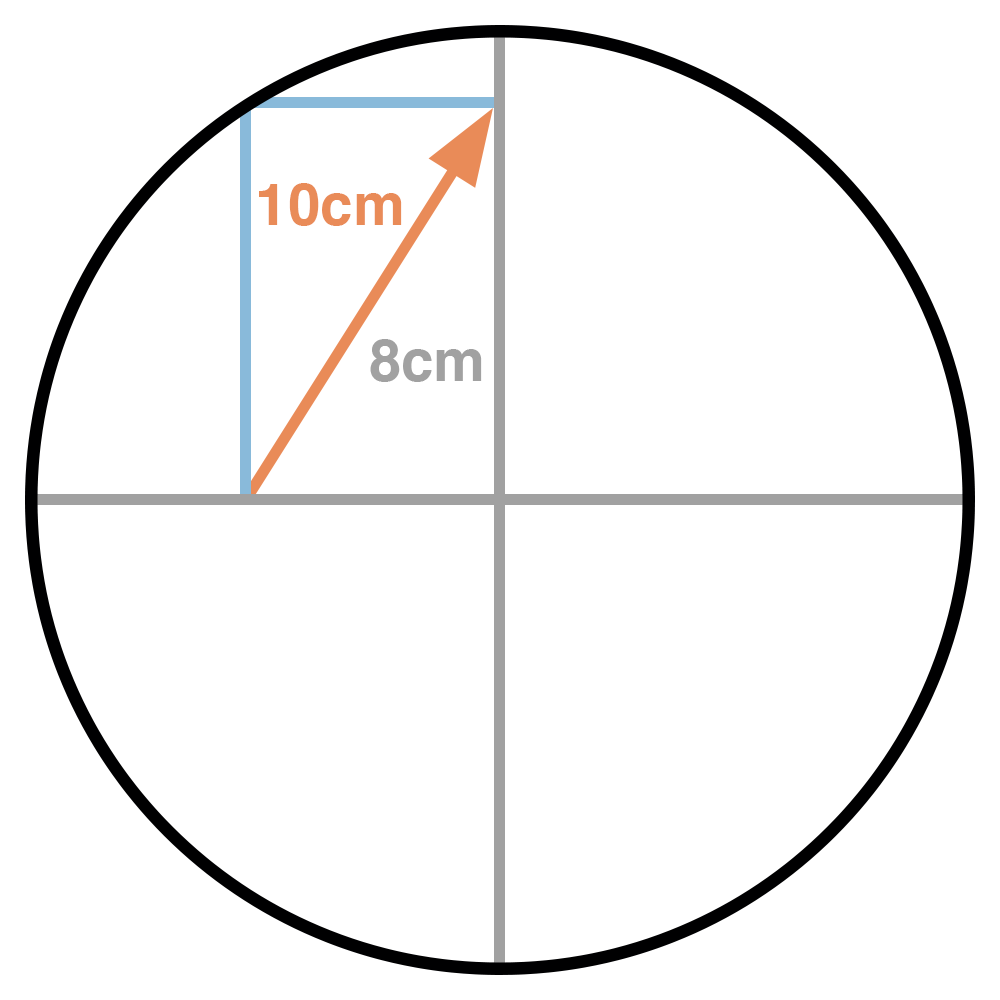# Circle Conundrum

Geometry Level 1If the area of the circle can be expressed as $A \pi \text{ cm}^2$, find the value of $A$.

A rectangle is drawn in a circle so that one vertex lies on the center point of the circle, and the opposite corner lies on the circumference. The height of the rectangle is $8\text{ cm}$, and its diagonal is $10\text{ cm}$.

×

Problem Loading...

Note Loading...

Set Loading...